# Theoretical Approaches to Machine Learning Early work eg

• Slides: 38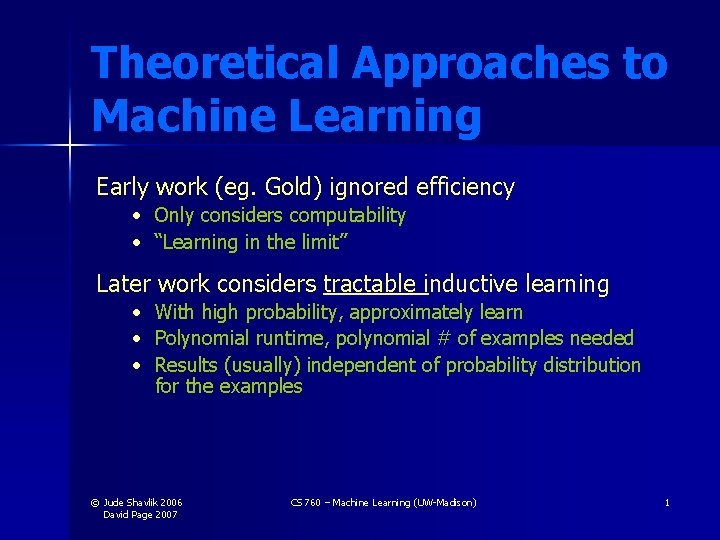Theoretical Approaches to Machine Learning Early work (eg. Gold) ignored efficiency • Only considers computability • “Learning in the limit” Later work considers tractable inductive learning • • • With high probability, approximately learn Polynomial runtime, polynomial # of examples needed Results (usually) independent of probability distribution for the examples © Jude Shavlik 2006 David Page 2007 CS 760 – Machine Learning (UW-Madison) 1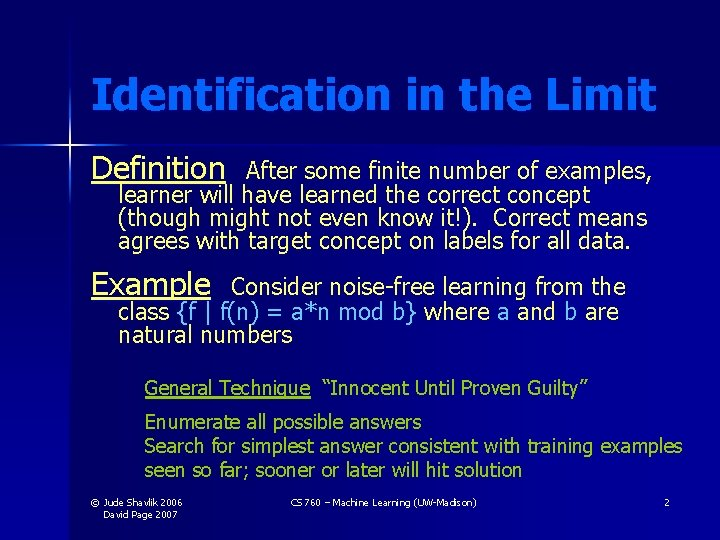Identification in the Limit Definition After some finite number of examples, learner will have learned the correct concept (though might not even know it!). Correct means agrees with target concept on labels for all data. Example Consider noise-free learning from the class {f | f(n) = a*n mod b} where a and b are natural numbers General Technique “Innocent Until Proven Guilty” Enumerate all possible answers Search for simplest answer consistent with training examples seen so far; sooner or later will hit solution © Jude Shavlik 2006 David Page 2007 CS 760 – Machine Learning (UW-Madison) 2Some Results (Gold) • Computable languages (Turing machines) can be learned in the limit using inference by enumeration. • If data set is limited to positive examples only, then only finite languages can be learned in the limit. © Jude Shavlik 2006 David Page 2007 CS 760 – Machine Learning (UW-Madison) 3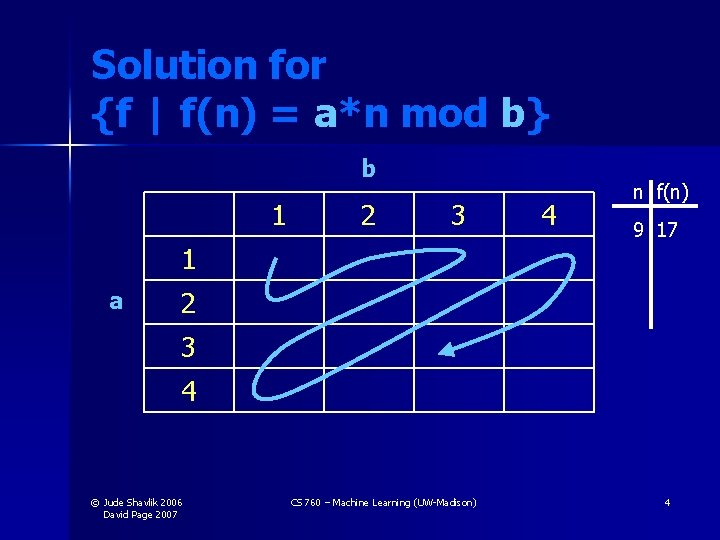Solution for {f | f(n) = a*n mod b} b 1 2 3 1 a 4 n f(n) 9 17 2 3 4 © Jude Shavlik 2006 David Page 2007 CS 760 – Machine Learning (UW-Madison) 4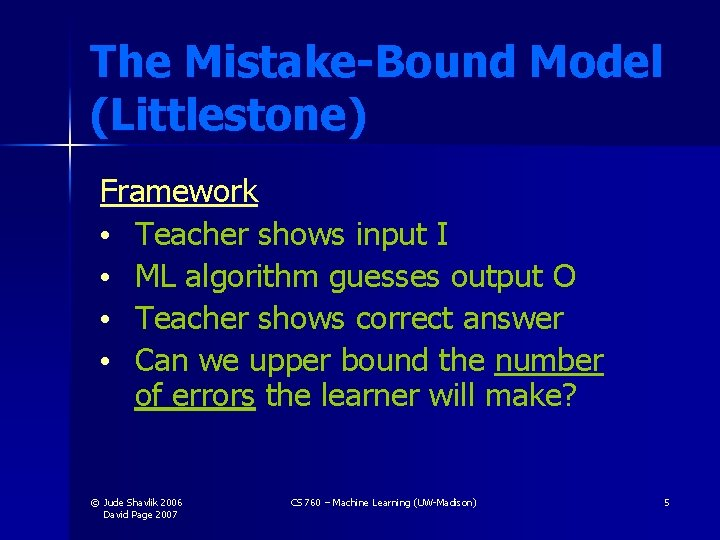The Mistake-Bound Model (Littlestone) Framework • Teacher shows input I • ML algorithm guesses output O • Teacher shows correct answer • Can we upper bound the number of errors the learner will make? © Jude Shavlik 2006 David Page 2007 CS 760 – Machine Learning (UW-Madison) 5The Mistake-Bound Model Example Learn a conjunct from N predicates and their negations • Initial h = p 1 ¬p 1 … pn ¬pn • For each + ex, remove the remaining terms that do not match © Jude Shavlik 2006 David Page 2007 CS 760 – Machine Learning (UW-Madison) 6The Mistake-Bound Model Worst case # of mistakes? 1+N • First + ex will remove N terms from hinitial • Each subsequent error on a + will remove at least one more term (never make a mistake on - ex’s) © Jude Shavlik 2006 David Page 2007 CS 760 – Machine Learning (UW-Madison) 7Equivalence Query Model (Angluin) Framework • ML algorithm guesses concept: is target equivalent to this guess? ) • Teacher either says “yes” or returns a counterexample (example labeled differently by target and guess) • Can we upper bound the number of errors the learner will make? • Time to compute next guess bounded by Poly(|data seen so far|) © Jude Shavlik 2006 David Page 2007 CS 760 – Machine Learning (UW-Madison) 8Probably Approximately Correct (PAC) Learning PAC learning (Valiant ’ 84) Given X C c C , © Jude Shavlik 2006 David Page 2007 domain of possible examples class of possible concepts to label X target concept correctness bounds CS 760 – Machine Learning (UW-Madison) 9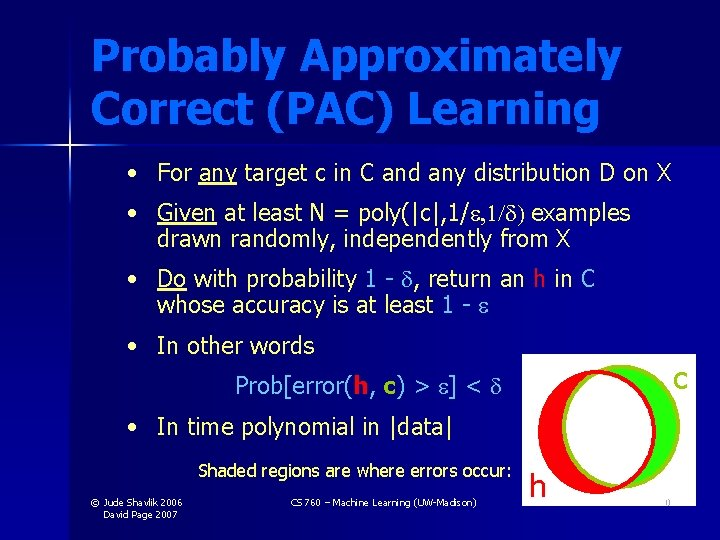Probably Approximately Correct (PAC) Learning • For any target c in C and any distribution D on X • Given at least N = poly(|c|, 1/ ) examples drawn randomly, independently from X • Do with probability 1 - , return an h in C whose accuracy is at least 1 - • In other words c Prob[error(h, c) > ] < • In time polynomial in |data| Shaded regions are where errors occur: © Jude Shavlik 2006 David Page 2007 CS 760 – Machine Learning (UW-Madison) h 10Relationships Among Models of Tractable Learning • Poly mistake-bounded with poly update time = EQ-learning • EQ-learning implies PAC-learning • Simulate teacher by poly-sized random sample; if all labeled correctly, say “yes”; otherwise, return incorrect example • On each query, increase sample size based on Bonferoni correction © Jude Shavlik 2006 David Page 2007 CS 760 – Machine Learning (UW-Madison) 11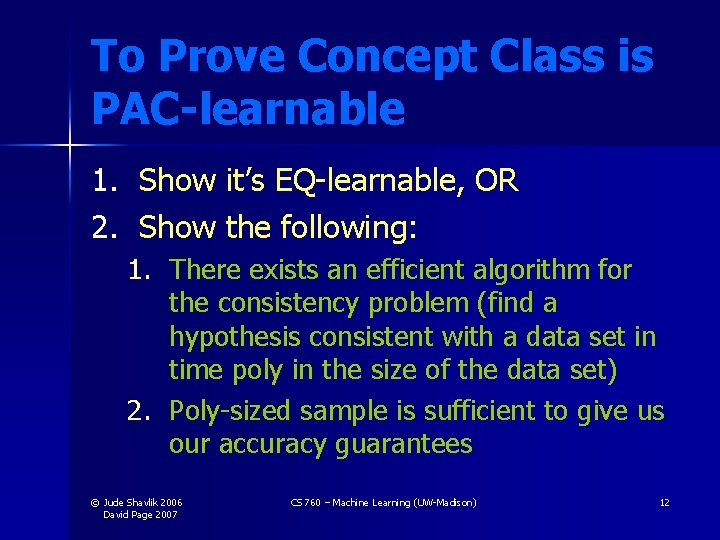To Prove Concept Class is PAC-learnable 1. Show it’s EQ-learnable, OR 2. Show the following: 1. There exists an efficient algorithm for the consistency problem (find a hypothesis consistent with a data set in time poly in the size of the data set) 2. Poly-sized sample is sufficient to give us our accuracy guarantees © Jude Shavlik 2006 David Page 2007 CS 760 – Machine Learning (UW-Madison) 12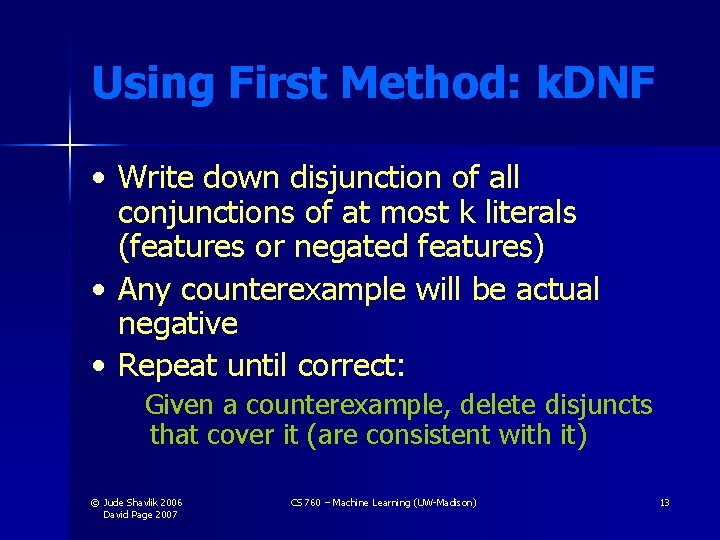Using First Method: k. DNF • Write down disjunction of all conjunctions of at most k literals (features or negated features) • Any counterexample will be actual negative • Repeat until correct: Given a counterexample, delete disjuncts that cover it (are consistent with it) © Jude Shavlik 2006 David Page 2007 CS 760 – Machine Learning (UW-Madison) 13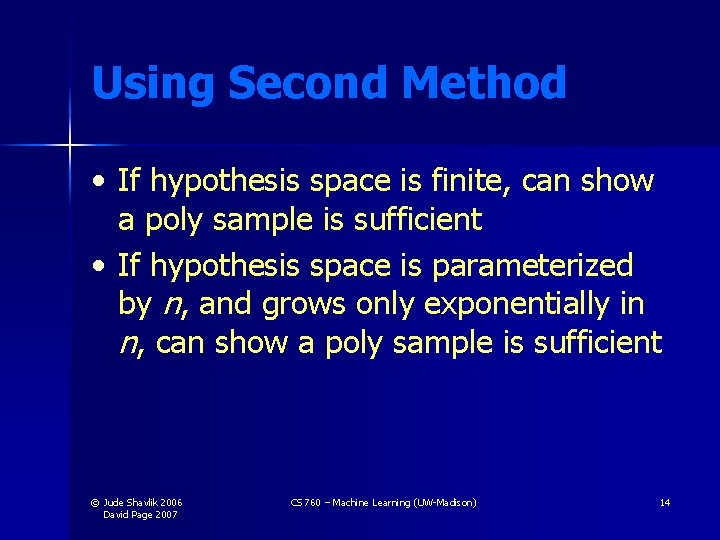Using Second Method • If hypothesis space is finite, can show a poly sample is sufficient • If hypothesis space is parameterized by n, and grows only exponentially in n, can show a poly sample is sufficient © Jude Shavlik 2006 David Page 2007 CS 760 – Machine Learning (UW-Madison) 14How Many Examples Needed to be PAC? • Consider finite hypothesis spaces • Let Hbad {h 1, …, hz} • The set of hypotheses whose (“testset”) error is > • Goal Eliminate all items in Hbad via (noise-free) training examples © Jude Shavlik 2006 David Page 2007 CS 760 – Machine Learning (UW-Madison) 15How Many Examples Needed to be PAC? How can an h look bad, even though it is correct on all the training examples? • • If we never see any examples in the shaded regions We’ll compute an N s. t. the odds of this are sufficiently low (recall, N = number of examples) c h © Jude Shavlik 2006 David Page 2007 CS 760 – Machine Learning (UW-Madison) 16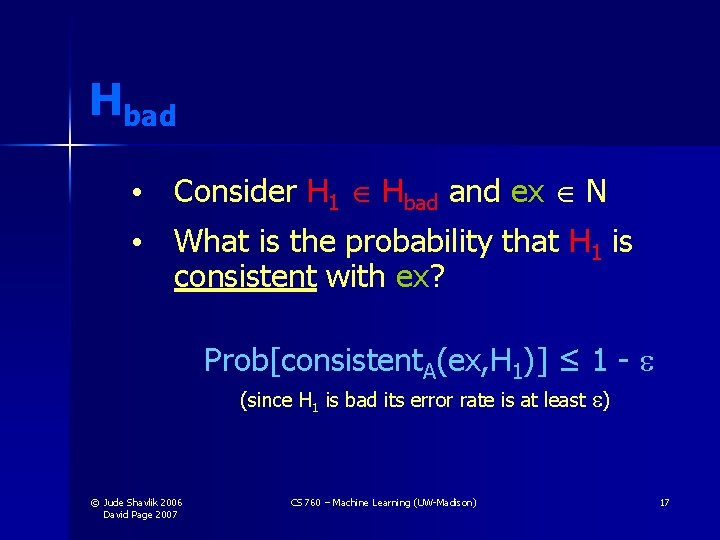Hbad • Consider H 1 Hbad and ex N • What is the probability that H 1 is consistent with ex? Prob[consistent. A(ex, H 1)] ≤ 1 - (since H 1 is bad its error rate is at least ) © Jude Shavlik 2006 David Page 2007 CS 760 – Machine Learning (UW-Madison) 17Hbad (cont. ) What is the probability that H 1 is consistent with all N examples? Prob[consistent. B(N, H 1)] ≤ (1 - )|N| (by iid assumption) © Jude Shavlik 2006 David Page 2007 CS 760 – Machine Learning (UW-Madison) 18Hbad (cont. ) What is the probability that some member of Hbad is consistent with the examples in N ? Prob[consistent. C(N, Hbad)] Prob[consistent. B(N, H 1) … consistent. B(N, Hz)] ≤ |Hbad| x (1 - )|N| // P(A B) P(A) + P(B) - P(A B) ≤ |H| x (1 - )|N| © Jude Shavlik 2006 David Page 2007 // Hbad H CS 760 – Machine Learning (UW-Madison) Ignore this in upper bound calc 19Solving for |N| We have Prob[consistent. C(N, Hbad)] ≤ |H| x (1 - )|N| < Recall that we want the prob of a bad concept surviving to be less than , our bound on learning a poor concept Assume that if many consistent hypotheses survive, we get unlucky and choose a bad one (we’re doing a worst-case analysis) © Jude Shavlik 2006 David Page 2007 CS 760 – Machine Learning (UW-Madison) 20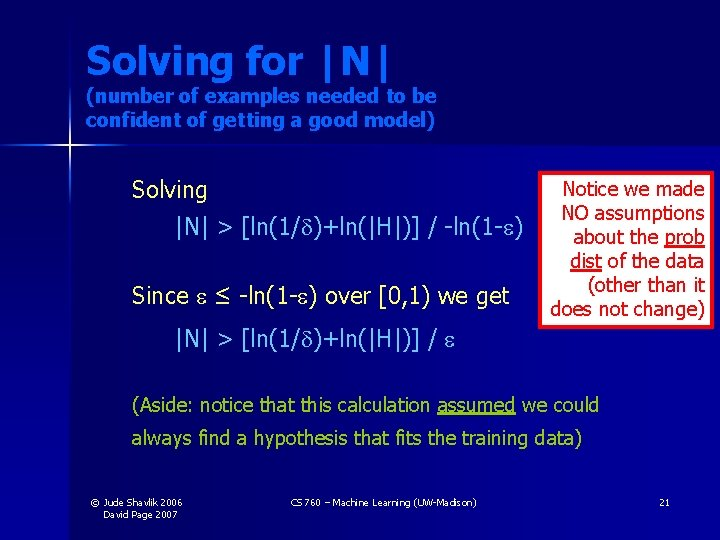Solving for |N| (number of examples needed to be confident of getting a good model) Solving |N| > [ln(1/ )+ln(|H|)] / -ln(1 - ) Since ≤ -ln(1 - ) over [0, 1) we get Notice we made NO assumptions about the prob dist of the data (other than it does not change) |N| > [ln(1/ )+ln(|H|)] / (Aside: notice that this calculation assumed we could always find a hypothesis that fits the training data) © Jude Shavlik 2006 David Page 2007 CS 760 – Machine Learning (UW-Madison) 21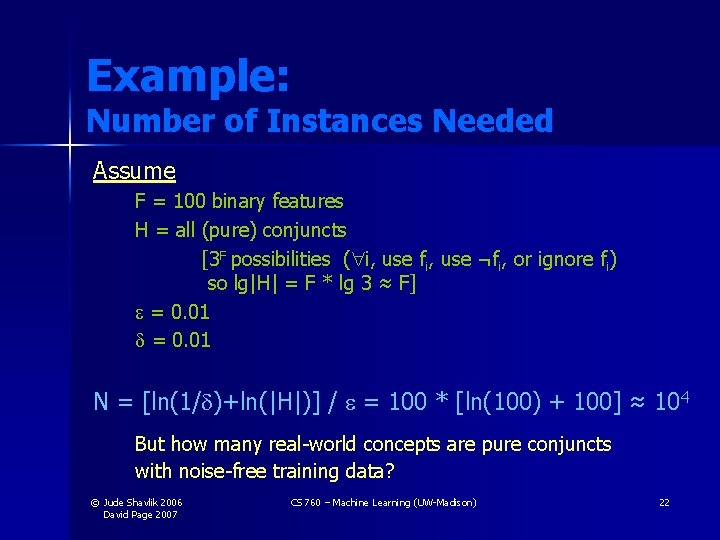Example: Number of Instances Needed Assume F = 100 binary features H = all (pure) conjuncts [3 F possibilities ( i, use fi, use ¬fi, or ignore fi) so lg|H| = F * lg 3 ≈ F] = 0. 01 N = [ln(1/ )+ln(|H|)] / = 100 * [ln(100) + 100] ≈ 104 But how many real-world concepts are pure conjuncts with noise-free training data? © Jude Shavlik 2006 David Page 2007 CS 760 – Machine Learning (UW-Madison) 22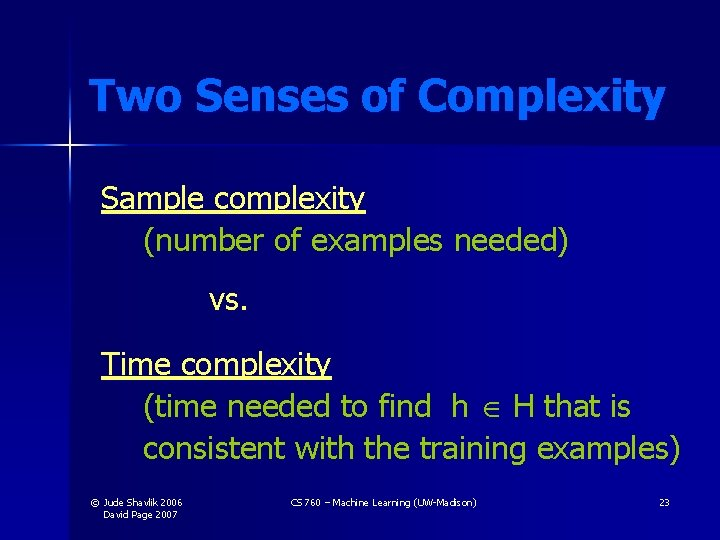Two Senses of Complexity Sample complexity (number of examples needed) vs. Time complexity (time needed to find h H that is consistent with the training examples) © Jude Shavlik 2006 David Page 2007 CS 760 – Machine Learning (UW-Madison) 23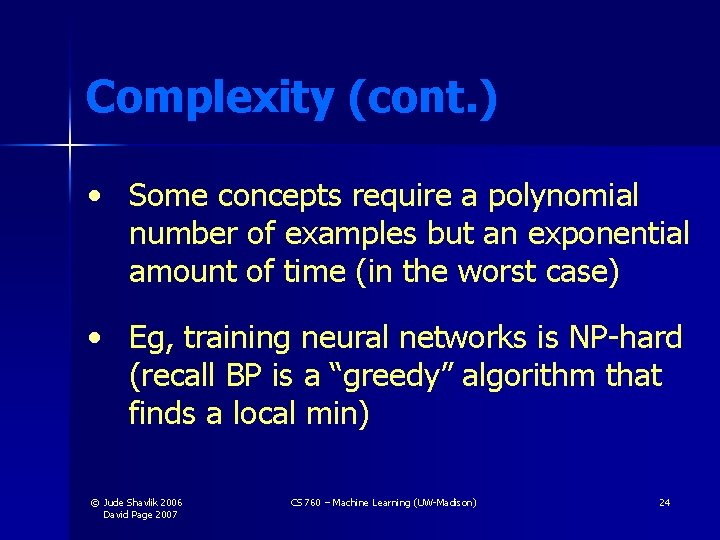Complexity (cont. ) • Some concepts require a polynomial number of examples but an exponential amount of time (in the worst case) • Eg, training neural networks is NP-hard (recall BP is a “greedy” algorithm that finds a local min) © Jude Shavlik 2006 David Page 2007 CS 760 – Machine Learning (UW-Madison) 24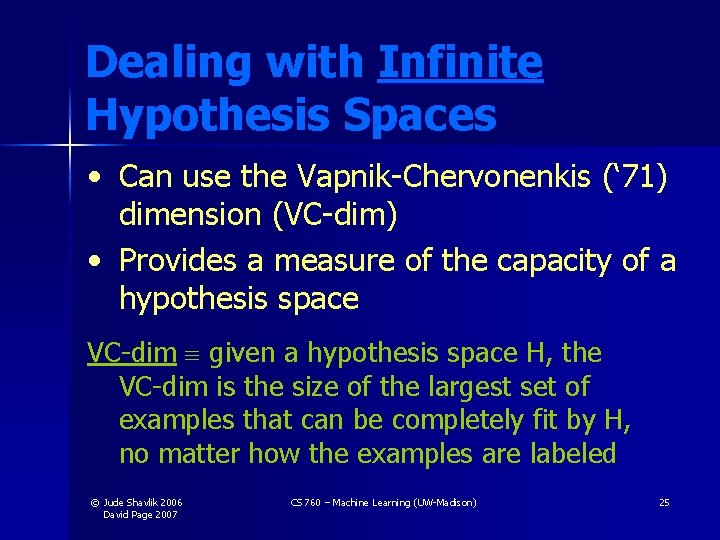Dealing with Infinite Hypothesis Spaces • Can use the Vapnik-Chervonenkis (‘ 71) dimension (VC-dim) • Provides a measure of the capacity of a hypothesis space VC-dim given a hypothesis space H, the VC-dim is the size of the largest set of examples that can be completely fit by H, no matter how the examples are labeled © Jude Shavlik 2006 David Page 2007 CS 760 – Machine Learning (UW-Madison) 25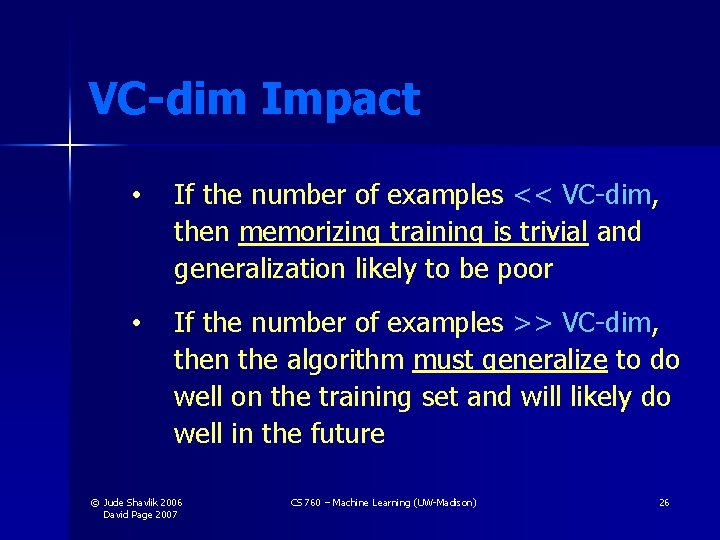VC-dim Impact • If the number of examples << VC-dim, then memorizing training is trivial and generalization likely to be poor • If the number of examples >> VC-dim, then the algorithm must generalize to do well on the training set and will likely do well in the future © Jude Shavlik 2006 David Page 2007 CS 760 – Machine Learning (UW-Madison) 26Samples of VC-dim Finite H VC-Dim ≤ log 2|H| (if d examples, 2 d different labelings possible, and must have 2 d ≤ |H| if all functions are to be in H) © Jude Shavlik 2006 David Page 2007 CS 760 – Machine Learning (UW-Madison) 27An Infinite Hypothesis Space with a Finite VC Dim H is set of lines in 2 D Can cover 1 ex no matter how labeled 1 © Jude Shavlik 2006 David Page 2007 CS 760 – Machine Learning (UW-Madison) 28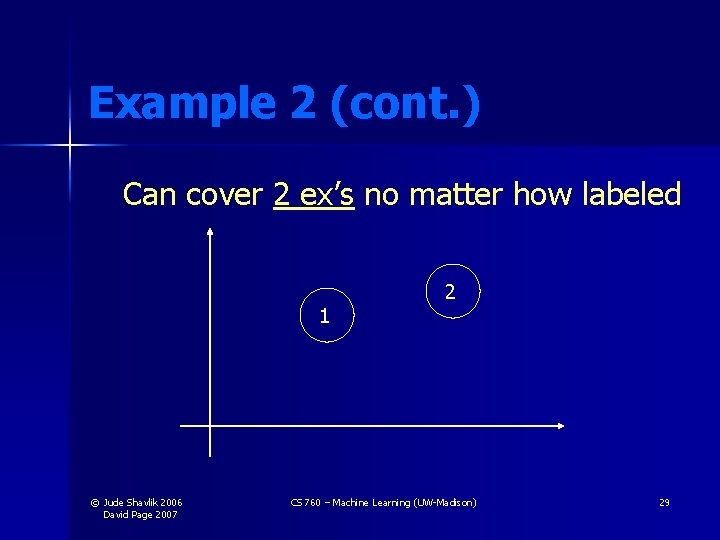Example 2 (cont. ) Can cover 2 ex’s no matter how labeled 1 © Jude Shavlik 2006 David Page 2007 2 CS 760 – Machine Learning (UW-Madison) 29Example 2 (cont. ) Can cover 3 ex’s no matter how labeled 1 2 3 © Jude Shavlik 2006 David Page 2007 CS 760 – Machine Learning (UW-Madison) 30Example 2 (cont. ) Cannot cover/separate if 1 and 4 are +, But 2 and 3 are – (our old friend, ex-or) 3 © Jude Shavlik 2006 David Page 2007 Notice |H| = ∞ but VC-dim = 3 2 1 4 CS 760 – Machine Learning (UW-Madison) For N-dimensions and N-1 dim hyperplanes, VC-dim = N + 1 31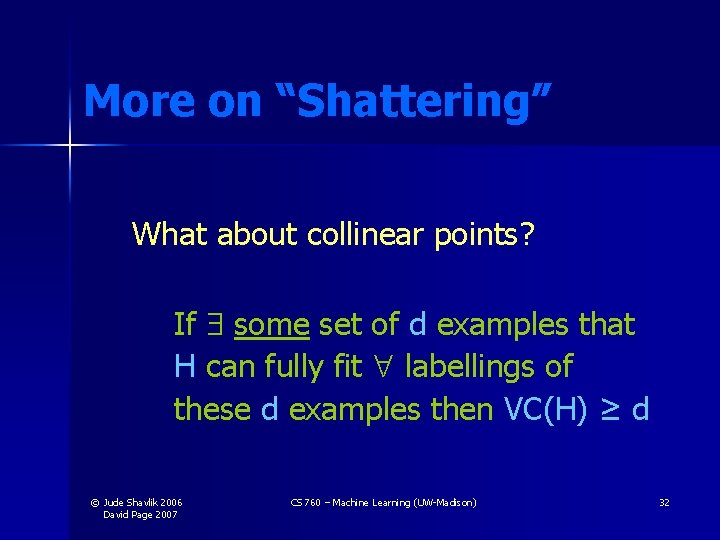More on “Shattering” What about collinear points? If some set of d examples that H can fully fit labellings of these d examples then VC(H) ≥ d © Jude Shavlik 2006 David Page 2007 CS 760 – Machine Learning (UW-Madison) 32Some VC-Dim Theorems Theorem H is PAC-learnable iff its VC-dim is finite Theorem Need, to be PAC, # of examples > 1/ max[4 ln(2/ ), 8 ln(13/ )VC-dim(H)] Theorem Any PAC algorithm needs at least (1/ [ln(1/ ) + VC-dim(H)]) examples [No need to memorize these for the exam] © Jude Shavlik 2006 David Page 2007 CS 760 – Machine Learning (UW-Madison) 33To Show a Concept is NOT PAC-learnable • Show the consistency problem is NPhard (hardness assumes P ≠ NP), OR • Show the VC-dimension grows at a rate not bounded by any polynomial © Jude Shavlik 2006 David Page 2007 CS 760 – Machine Learning (UW-Madison) 34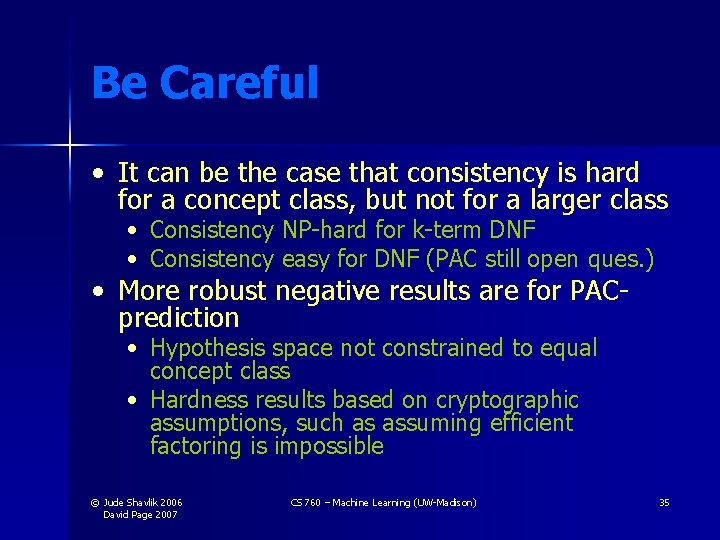Be Careful • It can be the case that consistency is hard for a concept class, but not for a larger class • Consistency NP-hard for k-term DNF • Consistency easy for DNF (PAC still open ques. ) • More robust negative results are for PACprediction • Hypothesis space not constrained to equal concept class • Hardness results based on cryptographic assumptions, such as assuming efficient factoring is impossible © Jude Shavlik 2006 David Page 2007 CS 760 – Machine Learning (UW-Madison) 35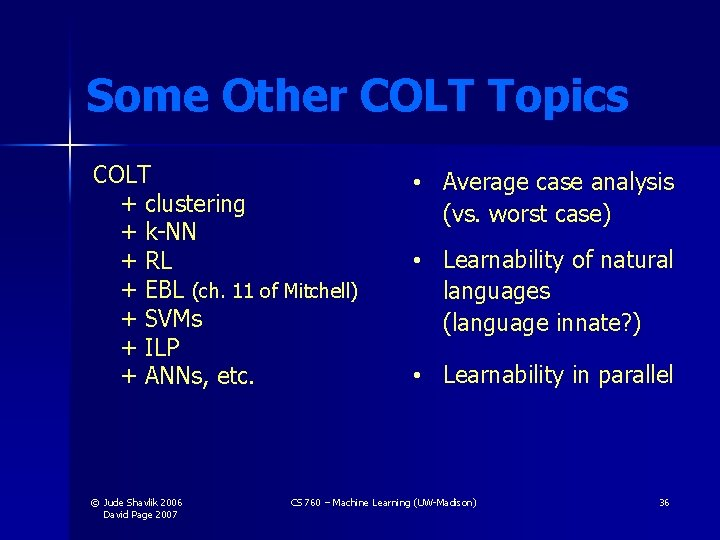Some Other COLT Topics COLT + clustering + k-NN + RL + EBL (ch. 11 of Mitchell) + SVMs + ILP + ANNs, etc. © Jude Shavlik 2006 David Page 2007 • Average case analysis (vs. worst case) • Learnability of natural languages (language innate? ) • Learnability in parallel CS 760 – Machine Learning (UW-Madison) 36Summary of COLT Strengths • • Formalizes learning task Allows for imperfections (e. g. and in PAC) Work on boosting excellent case of ML theory influencing ML practice Shows what concepts are intrinsically hard to learn (e. g. k-term DNF) © Jude Shavlik 2006 David Page 2007 CS 760 – Machine Learning (UW-Madison) 37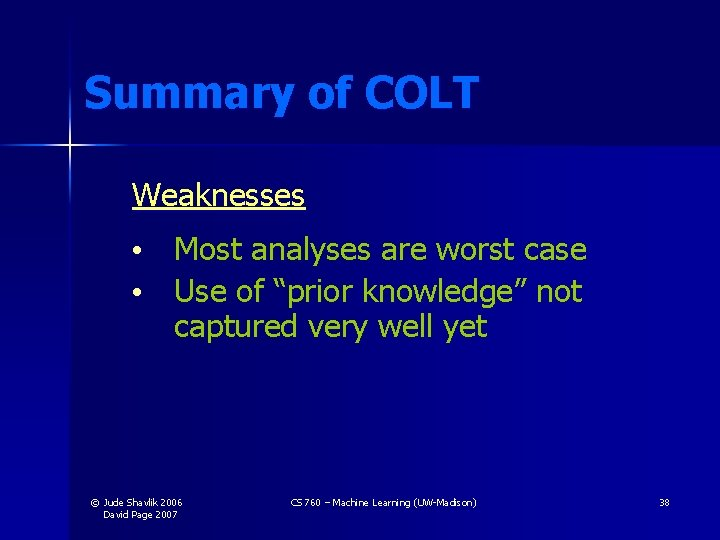Summary of COLT Weaknesses • Most analyses are worst case • Use of “prior knowledge” not captured very well yet © Jude Shavlik 2006 David Page 2007 CS 760 – Machine Learning (UW-Madison) 38